# 自学Python6.5-内置模块(re、collections、)

### 14. collections 模块

• 计数器（Counter），主要用来计数
• 双向队列（deque），双端队列，可以快速的从另外一侧追加和推出对象
• 默认字典（defaultdict），带默认值的字典
• 有序字典（OrderedDict）
• 可命名元组（namedtuple）　，生成可以使用名字来访问元素内容的tuple

14.1 计数器（Counter）

Counter作为字典dicit（）的一个子类用来进行hashtable计数，将元素进行数量统计，计数后返回一个字典，键值为元素，值为元素个数

 most_common(int) 按照元素出现的次数进行从高到低的排序，返回前int个元素的字典 elements 返回经过计算器Counter后的元素，返回的是一个迭代器 update 和set集合的update一样，对集合进行并集更新 substract 和update类似，只是update是做加法，substract做减法,从另一个集合中减去本集合的元素 iteritems 返回由Counter生成的字典的所有item iterkeys 返回由Counter生成的字典的所有key itervalues 返回由Counter生成的字典的所有value
from collections import Counter

li = ["a","b","c","a","b","b"]
d = {"1":3, "3":2, "17":2}

#1. Counter获取各元素的个数，返回字典
print ("Counter(s):", Counter(str))
print ("Counter(li):", Counter(li))
print ("Counter(d):", Counter(d))

#2. most_common(int)按照元素出现的次数进行从高到低的排序，返回前int个元素的字典
d1 = Counter(str)
print ("d1.most_common(2):",d1.most_common(2))

#3. elements返回经过计算器Counter后的元素，返回的是一个迭代器
print ("sorted(d1.elements()):", sorted(d1.elements()))
print ('''("".join(d1.elements())):''',"".join(d1.elements()))

#4. 若是字典的话返回value个key
d2 = Counter(d)
print("若是字典的话返回value个key:", sorted(d2.elements()))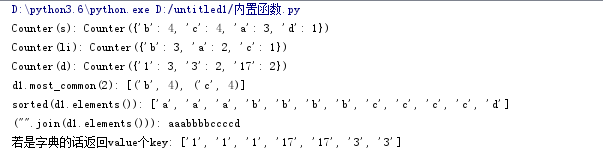14.2 双向队列（deque）

duque就是为了高效实现插入和删除操作的双向列表，适用于队列和栈。

• 堆栈：先进后出
• 队列queue：先进先出
#队列
import queue
q = queue.Queue()
q.put([1,2,3])
q.put(5)
q.put(6)
print(q)  #
print(q.get())
print(q.get())
print(q.get())
print(q.get())   # 取完3个数之后，阻塞
print(q.qsize()) # 取q的数值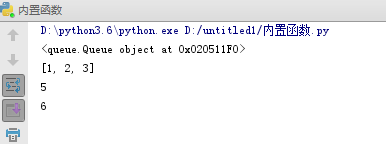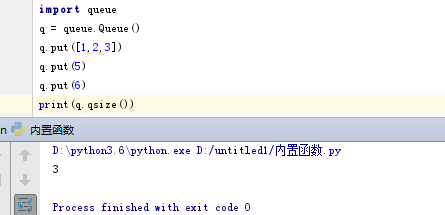# 队列
from collections import deque
dq = deque([1,2])
dq.append('a')   # 从后面放数据  [1,2,'a']
dq.appendleft('b') # 从前面放数据 ['b',1,2,'a']
dq.insert(2,3)    #['b',1,3,2,'a']
print(dq.pop())      # 从后面取数据 a
print(dq.pop())      # 从后面取数据 2
print(dq.popleft())  # 从前面取数据 b
print(dq)
 append 队列右边添加元素 appendleft 队列左边添加元素 clear 清空队列中的所有元素 count 返回队列中包含value的个数 extend 队列右边扩展，可以是列表、元组或字典，如果是字典则将字典的key加入到deque extendleft 同extend，在左边扩展 pop 移除并返回队列右边的元素 popleft 移除并返回队列左边的元素 remove（value） 移除队列第一个出现的元素 reverse 队列的所有元素进行反转 rotate（n） 对队列数进行移动14.3 默认字典（defaultdict）

from collections import defaultdict
d = defaultdict(lambda : 5)
print(d['k'])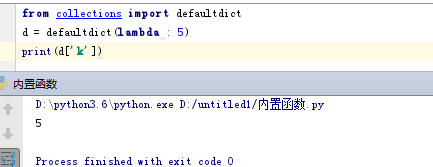14.4 有序字典（OrderedDict）

from collections import  OrderedDict
od = OrderedDict([('a', 1), ('b', 2), ('c', 3)])
print(od)  # OrderedDict的Key是有序的
print(od['a'])
for k in od:
print(k)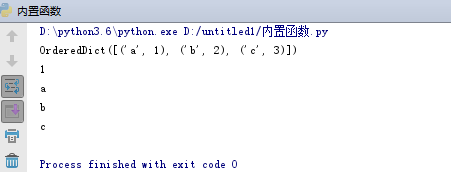14.5 可命名元组（namedtuple）

from collections import namedtuple
Point = namedtuple('point',['x','y','z'])
p1 = Point(1,2,3)
p2 = Point(3,2,1)
print(p1.x)
print(p1.y)
print(p1,p2)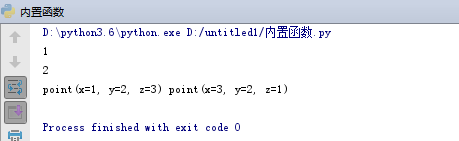#花色和数字
from collections import namedtuple
Card = namedtuple('card',['suits','number'])
c1 = Card('红桃',2)
print(c1)
print(c1.number)
print(c1.suits)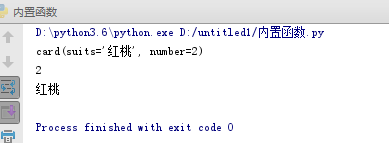### 15.  模块

posted on 2019-08-08 17:26  CARLOS_CHIANG  阅读(...)  评论(... 编辑 收藏